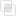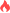python多进程中多个参数函数的使用

Python 多进程2021-09-13 10:08:20907浏览 · 0收藏 · 0评论1、在多参数函数，如果只想在多进程任务中依次取一个参数可迭代对象中的每个值，其他参数是固定的，使用偏函数来构建单参数函数。

2、不要用lambda函数代替偏函数，否则会报局部函数不能序列化的错误。

```from multiprocessing import Pool
from tqdm import tqdm
import numpy as np
import math
import time
from functools import partial

def func(x,y):
time.sleep(0.1)
return math.sin(x)+math.cos(y)

with Pool(processes = 20) as pool:
result = list(tqdm(pool.imap(partial(func,y = math.pi), np.linspace(0,2*math.pi,1000)), total=1000))```初识Python-Python的历史与优缺点Python中文分词工具Python中文分词的原理你知道吗？什么是网络协议python中的去除重复项的操作php多条件查询数据库的实现方法869

php去掉尖括号的方法1401

php查询某个字段的方法1381